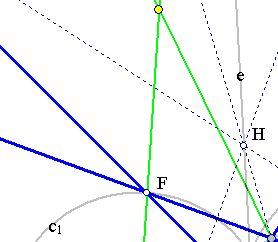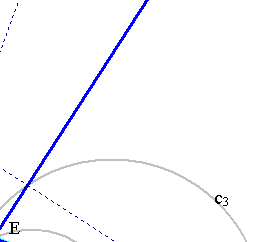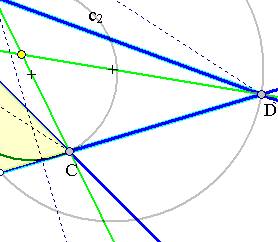##Gauss Bodenmiller Theorem

The circles c1, c2, c3 having diameters the diagonals BF, CE, AD of a complete quadrilateral ACDE are coaxal (i.e. belong to the same circle bundle i.e. they have pairwise the same radical axis e).In fact, the three circles pass from the end-points of the altitudes of triangle ABC. The same is true for the altitudes of triangle EBD. Thus (see Orthocenter2.html ), the orthocenters G, H of these two triangles belong to the radical axis of any two circles of the three. This proves the theorem. The argument uses the fact that the orthocenters of the four triangles ABC, EBD, FCD, FAE cannot be all identical. It implies also that these four orthocenters are on line e, the common radical axis of the bundle defined by the three circles.
The theorem has as a consequence the theorem of Newton (see Newton.html ). It can be also formulated in another way:
Given a triangle ABC and a line DEF intersecting its sides. The three circles having diameters the vertices of the triangle and the opposite intersection points BF, AD, CE are coaxal.

Newton.html
Miquel_Point.html
Orthocenter.html
Orthocenter2.html
OrthoRectangular.html
RectHypeCircumscribed.html
RectHypeThroughFourPts.html

### References

Johnson, R. A. Modern Geometry: An Elementary Treatise on the Geometry of the Triangle and the Circle. Boston, MA: Houghton Mifflin, 1929, p. 172.

 Produced with EucliDraw© http://users.math.uoc.gr/~pamfilos/